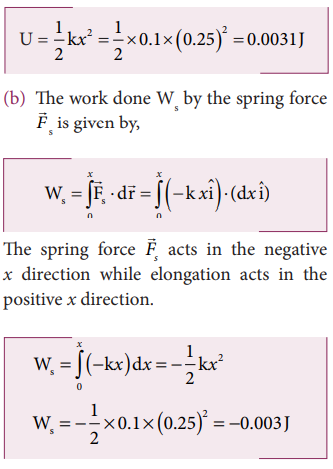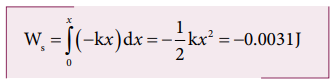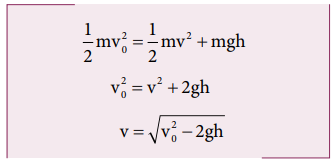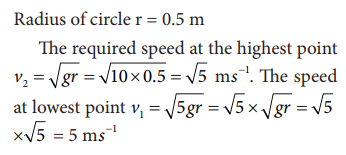Home | | Physics 11th std | Solved Example Problems for Energy

# Solved Example Problems for Energy

Physics : Work, Energy and Power : Solved Example Problems for Energy

## Solved Example Problems for  Kinetic energy

### Example 4.7

Two objects of masses 2 kg and 4 kg are moving with the same momentum of 20 kg m s-1.

a. Will they have same kinetic energy?

b. Will they have same speed?

### Solution

a. The kinetic energy of the mass is given byNote that KE1 ≠ KE2 i.e., even though both are having the same momentum, the kinetic energy of both masses is not the same. The kinetic energy of the heavier object has lesser kinetic energy than smaller mass. It is because the kinetic energy is inversely proportional to the mass (KE  1/m) for a given momentum.

b. As the momentum, p = mv, the two objects will not have same speed.

## Solved Example Problems for  Potential Energy

### Example 4.8

An object of mass 2 kg is taken to a height 5 m from the ground g = 10ms-2 .

a. Calculate the potential energy stored in the object.

b. Where does this potential energy come from?

c. What external force must act to bring the mass to that height?

d. What is the net force that acts on the object while the object is taken to the height ‘h’?

### Solution

a. The potential energy U =m g h = 2 × 10 × 5 = 100 J

Here the positive sign implies that the energy is stored on the mass.

b. This potential energy is transferred from external agency which applies the force on the mass.

c.d. From the definition of potential energy, the object must be moved at constant velocity. So the net force acting on the object is zero.## Solved Example Problems for  Elastic Potential Energy

Example 4.9

Let the two springs A and B be such that kA>kB. On which spring will more work has to be done if they are stretched by the same force?

### SolutionThe work done on the springs are stored as potential energy in the springs.kA>kB implies that UB>UA . Thus, more work is done on B than A.

### Example 4.10

A body of mass m is attached to the spring which is elongated to 25 cm by an applied force from its equilibrium position.

a. Calculate the potential energy stored in the spring-mass system?

b. What is the work done by the spring force in this elongation?

c. Suppose the spring is compressed to the same 25 cm, calculate the potential energy stored and also the work done by the spring force during compression. (The spring constant, k = 0.1 N m-1).

### Solution

The spring constant, k = 0.1 N m-1

The displacement, x = 25 cm = 0.25 m

a. The potential energy stored in the spring is given byNote that the potential energy is defined through the work done by the external agency. The positive sign in the potential energy implies that the energy is transferred from the agency to the object. But the work done by the restoring force in this case is negative since restoring force is in the opposite direction to the displacement direction.

c. During compression also the potential energy stored in the object is the same.Work done by the restoring spring force during compression is given byIn the case of compression, the restoring spring force acts towards positive x-axis and displacement is along negative x direction.## Solved Example Problems for  Conservative and nonconservative forces

### Example 4.11

Compute the work done by the gravitational force for the following casesSolution(As the displacement is in two dimension; unit vectors and are used)

a. Since the motion is only vertical, horizontal displacement component dx is zero. Hence, work done by the force along path 1 (of distance h).Therefore, the total work done by the force along the path 2 isNote that the work done by the conservative force is independent of the path.

### Example 4.12

Consider an object of mass 2 kg moved by an external force 20 N in a surface having coefficient of kinetic friction 0.9 to a distance 10 m. What is the work done by the external force and kinetic friction ? Comment on the result. (Assume g = 10 ms-2)

Solution

= 2 kg, d = 10 m, Fext = 20 N, k = 0.9. When an object is in motion on the horizontal surface, it experiences two forces.

a. External force, Fext = 20 N

b. Kinetic friction,

fk =μkmg = 0.9x(2)x10=18N.

The work done by the external force  Wext = Fs = 20x20 =200J

The work done by the force of kinetic friction Wk =fkd = (-18) x10=-180J Here the negative sign implies that the force of kinetic friction is  pposite to the direction of displacement.

The total work done on the object

Wtotal = Wext + Wk = 200 J – 180 J = 20 J .

Since the friction is a non-conservative force, out of 200 J given by the external force, the 180 J is lost and it can not be recovered.

## Solved Example Problems for  Law of conservation of energy

### Example 4.13

An object of mass 1 kg is falling from the height h = 10 m. Calculate

a. The total energy of an object at h =10 m

b. Potential energy of the object when it is at h = 4 m

c. Kinetic energy of the object when it is at h = 4 m

d. What will be the speed of the object when it hits the ground?

(Assume g =10 m s-2)

### Solution

a. The gravitational force is a conservative force. So the total energy remains constant throughout the motion. At =10 m, the total energyis entirely potential energy.b. The potential energy of the object at = 4 m isc. Since the total energy is constant throughout the motion, the kinetic energy at h = 4 m must be KE = E - U = 100 - 40 = 60J

Alternatively, the kinetic energy could also be found from velocity of the object at 4 m. At the height 4 m, the object has fallen through a height of 6 m.

The velocity after falling 6 m is calculated from the equation of motion,d. When the object is just about to hit the ground, the total energy is completely kinetic and the potential energy, U = 0.### Example 4.14

A body of mass 100 kg is lifted to a height 10 m from the ground in two different ways as shown in the figure. What is the work done by the gravity in both the cases? Why is it easier to take the object through a ramp?Solution

m = 100 kg, h = 10 m

Along path (1):

The minimum force F1 required to move the object to the height of 10 m should be equal to the gravitational force, F1 mg = 100 x 10 = 1000 N

The distance moved along path (1) is, =10 m

The work done on the object along path (1) is

W = Fh = 1000 x 10 = 10,000 J

Along path (2):

In the case of the ramp, the minimum force F2 that we apply on the object to take it up is not equal to mg, it is rather equal to mg sinθ .(mg sin < mg) .

Here, angle θ = 30o

Therefore, F2  = mg sinθ = 100 × 10 ×  sin30o = 100 × 10 × 0.5 = 500N

Hence,  (mg sinθ < mg)

The path covered along the ramp is,

l = h/sin30 = 10/0.5 =20m

The work done on the object along path  (2) is, W = F2 l = 500 × 20 = 10,000 J

Since the gravitational force is a conservative force, the work done by gravity on the object is independent of the path taken.

In both the paths the work done by the gravitational force is 10,000 J

Along path (1): more force needs to be applied against gravity to cover lesser distance .

Along path (2): lesser force needs to be applied against the gravity to cover more distance.

As the force needs to be applied along the ramp is less, it is easier to move the object along the ramp.

### Example 4.15

An object of mass m is projected from the ground with initial speed v0.

Find the speed at height h.

### Solution

Since the gravitational force is conservative; the total energy is conserved throughout the motion.Final values of potential energy, kinetic energy and total energy are measured at the height h.

By law of conservation of energy, the initial and final total energies are the same.Note that in section (2.11.2) similar result is obtained using kinematic equation based on calculus method. However, calculation through energy conservation method is much easier than calculus method.

### Example 4.16

An object of mass 2 kg attached to a spring is moved to a distance x =10 m from its equilibrium position. The spring constant =1 N m-1and assume that the surface is frictionless.

a. When the mass crosses the equilibrium position, what is the speed of the mass?

b. What is the force that acts on the object when the mass crosses the equilibrium position and extremum position x = ± 10 m.

### Solution

a. Since the spring force is a conservative force, the total energy is constant. At x =10 m, the total energy is purely potential.When the mass crosses the equilibrium position x = 0 , the potential energyThe entire energy is purely kinetic energy at this position.b. Since the restoring spring force is F = - kx, when the object crosses the equilibrium position, it experiences no force. Note that at equilibrium position, the object moves very fast. When the object is at x = +10 m (elongation), the force F = - k x

F = - (1) (10) = - 10 N. Here the negative sign implies that the force is towards equilibrium i.e., towards negative x-axis and when the object is at x = - 10 (compression), it experiences a forces F = - (1) (- 10) = +10 N. Here the positive sign implies that the force points towards positive x-axis.

The object comes to momentary rest at x = ±10m even though it experiences a maximum force at both these points.

## Solved Example Problems for  Motion in a vertical circle

### Example 4.17

Water in a bucket tied with rope is whirled around in a vertical circle of radius 0.5 m. Calculate the minimum velocity at the lowest point so that the water does not spill from it in the course of motion. (g = 10 ms-2)

### SolutionStudy Material, Lecturing Notes, Assignment, Reference, Wiki description explanation, brief detail
11th Physics : UNIT 4 : Work, Energy and Power : Solved Example Problems for Energy |